This paper is part 3 in the Ultra-Fine Line Design Guide series.

Conventional differential pairs in circuit boards have adjacent return planes which strongly affect their differential impedance. Due to constraints in keeping the total board thickness under a maximum value and using linewidths larger than 4 mils, the coupling between the two lines in a differential pair is usually much less than the coupling of each line to the adjacent plane.

With the recent introduction of the Averatek Semi-Additive Process (A-SAP™) process, linewidths under 1 mil are possible using the same fabrication processing equipment as for traditional 4 mil wide lines. This opens up a new design space where the coupling between the traces can be much larger than the coupling to the adjacent plane. This means a new set of design rules must be applied for ultra-fine line differential pairs.

### Methodology to Explore Fine Line Geometries

In a previous paper, we introduced a methodology to explore design space of a differential pair to find the optimized features and achieve a target impedance with other parameters as a constraint. In addition, we introduced the span of a differential pair as a new metric to describe the extent of its boundaries. The geometrical features of a differential pair are defined in Figure 1.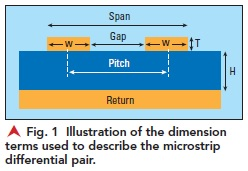In this paper, we use this methodology to explore the design space for a differential pair when the return plane is far enough away that coupling to it is negligible. This is a possible option only for very fine line traces with a small span, which is the realm of ultra-fine line widths.

### When Does the Coupling to the Adjacent Plane Not Matter?

The ultra-fine line capability of the A-SAP™ process enables an interesting configuration for differential pairs with much higher coupling between the traces than to an adjacent plane. This is the configuration in a CAT5 twisted pair cable, for example.

As the coupling between two traces increases, there is a point at which the coupling to an adjacent plane reduces to an insignificant level. One way to picture this is to consider the return current distribution in the adjacent return plane for a differential signal.

When the two traces are separated by their line width, and the return plane is located a distance away to result in 100 ohm differential impedance, the return currents in the plane of each line in the differential pair is well localized under each trace, separate and succinct. It is not true that the return current of one line is carried by the other. The return current for each line is carried in the plane, spatially separated; this is shown in Figure 2a.

However, when the plane is moved farther away, the differential impedance increases, and the return currents for a differential signal begin to overlap and cancel out. When the plane is as far away as twice the gap separation, some of the return currents from the two lines have canceled out and the return current distribution in the plane is reduced as shown in Figure 2b.

When the return plane is so far away that all the return currents of the two lines completely overlap in the plane, there is no return current in the plane and the plane can be removed with no impact on the differential impedance. This is shown in the Figure 2c, where the height is 15 mils. In this case, it is absolutely true that the return current of one line is carried by the other. And, in this situation, the plane has no impact on the differential impedance of the pair.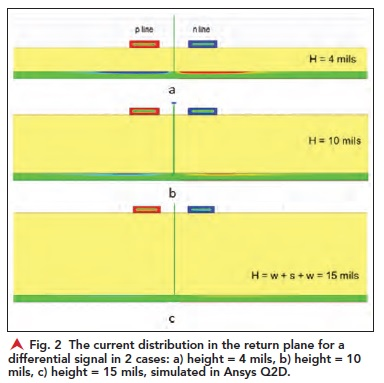Using the methodology developed previously, we can explore this design space to evaluate how far away the plane needs to be so that it has no impact on the differential impedance of the pair. In this case, the differential impedance is only about the coupling between the two lines in the pair.

In this analysis, the line width and the space between the two traces were fixed and the differential impedance was calculated using the built in 2D field solver in Keysight’s ADS as the trace height above the return place, h, was increased. Figure 3 is a plot of the differential impedance vs dielectric thickness (h) for a 1 oz trace thicknesses where the trace width was fixed at 2 mils and the gap separation was 6 mils. This would be an uncoupled differential pair if the plane was very close.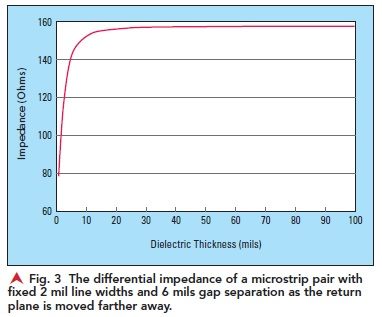This analysis shows that when the dielectric thickness is very large, the differential impedance is completely independent of the distance to the plane (h).  The limiting impedance is only about the conductor thickness – the line width and gap separation between the two lines. This is the case when the plane is so far away that the coupling between the two lines is much larger than the coupling to the plane below.

Using this limiting value of the differential impedance when the plane is very far away allows us to plot the relative impact on the impedance from the distance to the bottom plane, h. The results showing the differential impedance for a span of 10 mils, with 1 oz copper traces as the dielectric thickness, h, increases, are shown in Figure 4.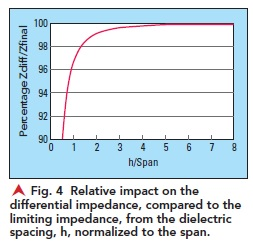To achieve 97% of the final differential impedance, the plane needs to be at least one span away. This analysis was repeated for spans of 4 mils, 10 mils, and 50 mils. For each span, the limiting impedance was calculated and used to normalize the differential impedance. Likewise, the span for each case was used to normalize the dielectric thickness. This analysis is shown in Figure 5.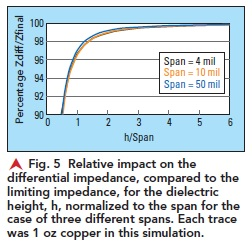Surprisingly, a dielectric height to the adjacent plane equal to the span of the differential pair is a good metric for how far away the plane needs to be to have all the return currents overlapping and the plane play no role at all. The span is a rough measure of the extent of the fringe field lines from the two lines in the pair.

This is the origin of a very powerful rule of thumb: in order to have a differential pair so tightly coupled that an adjacent plane does not affect its differential impedance, the plane should be farther away than the span of the pair.

This rule of thumb is an important guideline to identify when a differential pair’s impedance is independent of any return plane. For example, if the span of a differential pair is 5 mils, then a return plane more than 5 mils below the traces will have no impact on its differential impedance. In this case, the differential impedance of the pair is only related to their conductor thickness, line width, and spacing.

This rule of thumb also applies to thin and thick conductors. Figure 6 shows the same analysis for the relative differential impedance for a span of 4 mils, line width of 1 mil for each trace, and fixed gap separation of 2 mils, using conductor thicknesses of 0.5 oz to 3.5 oz copper traces. In this extreme environment, the differential impedance has achieved its nearly final value with a dielectric thickness of about 1 span for all conductor thicknesses.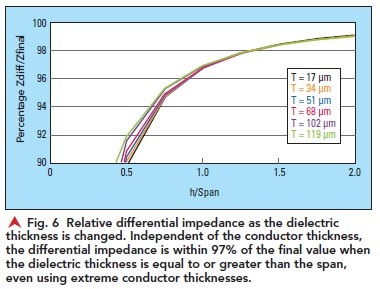This rule of thumb is surprisingly robust. It applies to 1 mil wide traces and ½ oz thickness, or an aspect ratio of trace thickness to line width of about 0.5, all the way to extreme aspect ratios of almost 5.

### A New Behavior Emerges in the Ultra-Fine Line Regime

This rule of thumb identifies when the design constraints eliminate the impact of the return plane on the differential impedance.

In this special case, when the return plane is moved so far away that there is no coupling to it, the differential impedance of the pair is determined only by the line-to-line coupling. Figure 7 shows that as the line width decreases, keeping the gap separation equal to the line width, the differential impedance decreases as well.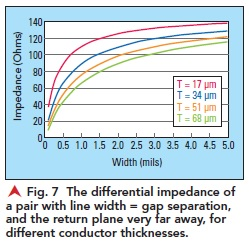Figure 7 shows that for a conductor thickness of even 2 oz copper or 68 micron, and line widths wider than 5 mils; the regime of conventional circuit board technology, the differential impedance, determined only from the line-to-line coupling, is much greater than 100 ohms. In order to achieve a target impedance of 100 ohms, in conventional technology, coupling to the adjacent plane is required to bring the differential impedance down to 100 ohms. Having an adjacent plane is a critical feature to achieve 100 ohms in conventional board technology. Otherwise, the differential impedance will always be larger than 100 ohms.

Figure 7 also shows that as the linewidth decreases, the differential impedance continues to drop. The coupling between the traces from their adjacent side walls transitions from a second or third order effect to a first order effect. In this special case of line width equaling gap separation, the trace thickness to the line width is about 1, and the differential impedance is about 100 ohms, without an adjacent return plane.

This is an important observation for high aspect ratio, ultra-fine lines. The additional fringe field coupling between the taller side walls can provide enough coupling to bring the differential impedance of a pair closer to 100 ohms. In the special case of traces with an aspect ratio of 1, with the line width equaling the gap separation equaling the conductor thickness – when the plane is very far away, and the differential pair is sitting on a relatively thick dielectric slab – the differential impedance is coincidently about 100 ohms.  This behavior is shown in the simulation in Figure 8.